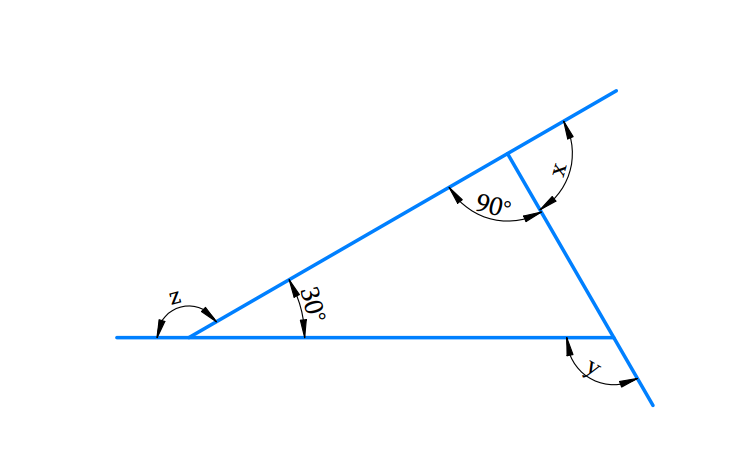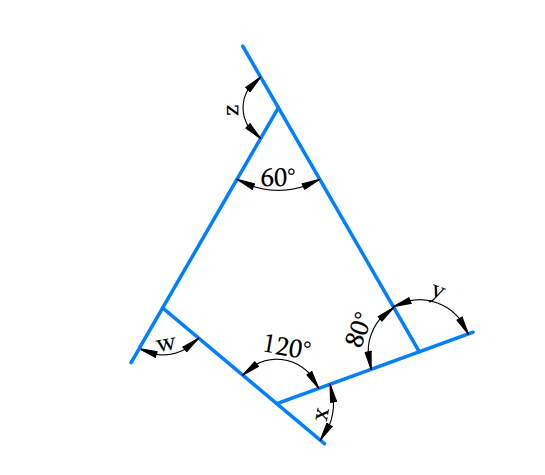# Ex.3.1 Q7 Understanding Quadrilaterals Solution - NCERT Maths Class 8

Go back to  'Ex.3.1'

## Question

a) Find $$x +y +z$$b) Find $$x +y +z +w$$## Text Solution

What is Known?

The sum of the measures of all the interior angles of a quadrilateral is $$360^\circ$$ and that of a triangle is $$180^\circ$$

What is Unknown?

Angle$$x, y, z$$ and $$w$$ in the above figures $$a$$ and $$b$$

Reasoning:

The unknown angles can be estimated by using the angle sum property of a quadrilateral and triangle accordingly.

Steps:

(a) Find $$x +y +z$$Sum of linear pair of angles is$$= {180^{\rm{o}}}$$

\begin{align}x + {90^{\rm{o}}} & = {180^{\rm{o}}}{\text{ (Linear pair)}}\\x & = {180^{\rm{o}}} - {90^{\rm{o}}}\\x & = {90^{\rm{o}}}\end{align}

And

\begin{align}z + 30^{\rm{o}} &= {180^{\rm{o}}}{\rm{ }}({\text{Linear pair}})\\z & = 180^{\rm{o}} - {30^{\rm{o}}}\\z &= {150^{\rm{o}}}\end{align}

And

\begin{align}y & = {90^{\rm{o}}} + {30^{\rm{o}}}{\text{ (Exterior angle theorem)}}\\y & = {120^{\rm{o}}}\end{align}

\begin{align}x + y + z & = {90^{\rm{o}}} + {120^{\rm{o}}} + {150^{\rm{o}}}\\& = {360^{\rm{o}}}\end{align}

(b)Find $$x +y +z$$The sum of the measures of all the interior angles of a quadrilateral is $$360^\circ.$$

Using the angle sum property of a quadrilateral,

Let $$n$$ is the fourth interior angle of the quadrilateral.

\begin{align}6{{0}^{\text{o}}}+8{{0}^{o}}+12{{0}^{o}}+n &=36{{0}^{o}} \\26{{0}^{o}}+n& =36{{0}^{o}} \\n& =36{{0}^{o}}-26{{0}^{o}} \\n& =10{{0}^{o}} \\\end{align}

sum of linear pair of angles is $$= {180^{\rm{o}}}$$

\begin{align}w + 100^\circ & = 180^\circ {\rm{ }} & \ldots \left( 1 \right)\\x + 120^\circ & = 180^\circ & \ldots \left( 2 \right)\\y + 80^\circ & = 180^\circ & \ldots \left( 3 \right)\\z + 60^\circ & = 180^\circ & \ldots \left( 4 \right)\end{align}

Adding equation ($$1), (2), (3)$$and $$(4),$$

\begin{align}w{\rm{ }} + {\rm{ }}100^\circ + x + 120^\circ + y + 80^\circ + z + 60^\circ & = {\rm{ }}180^\circ +180^\circ + 180^\circ + 180^\circ \\w + x + y + z + 360^\circ &= 720^\circ \\w{\rm{ }} + x + y{\rm{ }} + z &= 720^\circ - 360^\circ \\w + {\rm{ }}x + y + {\rm{ }}z &= 360^\circ\end{align}

The sum of the measures of the external angles of any polygon is $$360^\circ$$ .

Learn from the best math teachers and top your exams

• Live one on one classroom and doubt clearing
• Practice worksheets in and after class for conceptual clarity
• Personalized curriculum to keep up with school